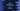# Introduction to Queue in Dart and its methods## How to implement Queue in dart:

The dart:collection library provides a class Queue to implement the Queue data structure. It also provides a couple of methods to iterate, add, remove items and a few properties to access different states of a Queue. In this post, we will learn how to use this class and its methods and properties with examples.

### Constructor to create a Queue object:

The Queue class provides three constructors to create one object:

#### Queue()

This is the default constructor, it creates one queue.

#### Queue.from(Iterable it)

By using this constructor, we can create one Queue from a given iterable. It will include the elements of the iterable to the Queue.

#### Queue.of(Iterable it)

It will create a Queue from the elements of the Iterable it.

### Properties of Queue:

The following properties are available for a Queue:

#### first:

It returns the first element of the Queue.

#### last:

It returns the last element of the Queue.

#### hashCode:

It returns the hash code of the queue object.

#### isEmpty:

It returns one boolean value defining if the queue is empty or not.

#### isNonEmpty:

It returns one boolean defining if the queue is not empty or empty.

#### iterator:

It returns one iterator to iterate over the elements of the queue.

#### length:

It returns an integer value holding the total number of elements in the queue.

#### single:

It checks if the queue has only one element or not and returns that element if yes.

#### runtimeType:

The runtime type of the queue object.

### Methods of Queue:

The following methods are defined in the Queue class:

It adds one element e at the end of the queue.

This method adds all the elements of the iterable to the end of the queue.

It adds the element to the start of the queue.

It adds the element to the end of the queue.

#### clear() -> void

This method removes all elements from the queue.

#### remove(Object o) -> bool

This method removes one instance of the object o from the queue.

#### removeFirst() -> E

This method removes the first element of the queue and returns this.

#### removeLast() -> E

This method removes the last element of the queue and returns that element.

#### removeWhere(bool fn(E e)) -> void

This method takes one function and removes all elements matched by the function from the queue.

#### retainWhere(bool fn(E e)) -> void

This method removes all elements not matched by the function fn.

### Inherited methods:

Other than the above methods, there are a couple of inherited methods of Queue:

#### any(bool test(E e)) -> bool

This method can be used to check if any element of the queue satisfies the provided function or not.

#### elementAt(int i) -> E

This method returns the element at index i of the queue.

#### contains(Object? e) -> bool

This method checks if the queue contains any element equal to e or not.

#### every(bool fn(E e)) -> bool

This method takes one function and checks if every element satisfies the function or not.

#### firstWhere(bool fn(E e), {E orElse()?}) -> E

Returns the first element of the queue satisfied by the given predicate.

#### expand(Iterable toElements(E e)) -> Iterable

Expands each element of the queue to zero or more elements.

#### fold(T initialValue, T combine(T previousValue, E element)) → T

Reduce the queue to a single value by combining each element iteratively.

#### followedBy(Iterable o) → Iterable

Get a lazy concatenation of this queue and another queue.

#### forEach(void action(E element)) → void

Use this to iterate over the queue elements.

#### join([String separator = ""]) → String

Get a string by concatenating the elements of the queue.

#### lastWhere(bool fn(E element), {E orElse()?}) → E

Get the last element that satisfies the provided predicate.

#### map(T toElement(E e)) → Iterable

Get the elements of the queue.

#### noSuchMethod(Invocation invocation) → dynamic

This method is invoked if we try to access a non-existent property or method.

#### reduce(E combine(E value, E element)) → E

Reduce the queue to a single value by iterating and combining the elements with the help of the combine function.

#### singleWhere(bool fn(E element), {E orElse()?}) → E

Get the element that is satisfied by the function fn.

#### skip(int n) → Iterable

Get one iterable with all the elements skipped by the first n elements.

#### skipWhile(bool fn(E value)) → Iterable

Get one iterable by skipping the elements satisfied by the function fn.

#### take(int n) → Iterable

Get a lazy iterable of the first n elements of the queue.

#### takeWhile(bool fn(E value)) → Iterable

Get a lazy iterable of the elements satisfying the function fn.

#### toList({bool growable = true}) → List

Get one List with queue elements.

#### toSet() → Set

Get one Set with the queue elements.

#### toString() → String

Get the string representation.

#### where(bool fn(E element)) → Iterable

It returns one lazy iterable with all the elements satisfies the predicate.

#### whereType() → Iterable

It returns one new lazy iterable.